# Motivation

When working with data, it is often the case that one is dependent on certain data types. Either to be able to work with it correctly or that results can be evaluated without errors. Unfortunately this is not always the case when for example the data is from a third party and simply has the wrong format (for example numbers represented as text). To deal with that, ONE DATA has the Data Type Conversion Processor.

# Overview

The Data Type Conversion Processor takes a given input and converts it, if possible, to the specified data type. The possible data types are stringdatetimedouble and integer If there are problems converting the given value, a fallback value can be specified that is returned instead, so the result is not empty.

# Input

The Processor takes any table as input.

# Configuration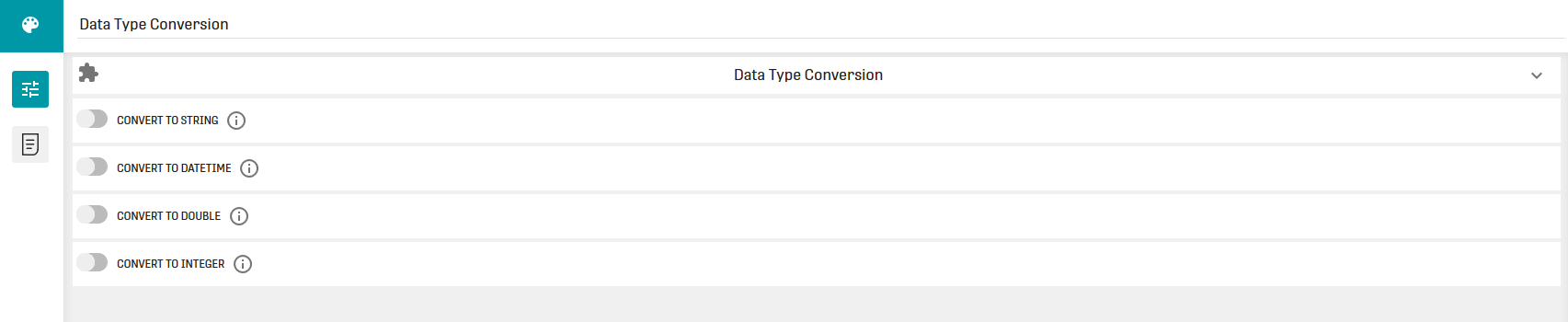The configuration of this processor gives us four different possibilities for data type conversion. For each option you can specify columns from the input value, that should be converted to a certain type. The configuration of the different options is described in the following section.

### Convert to String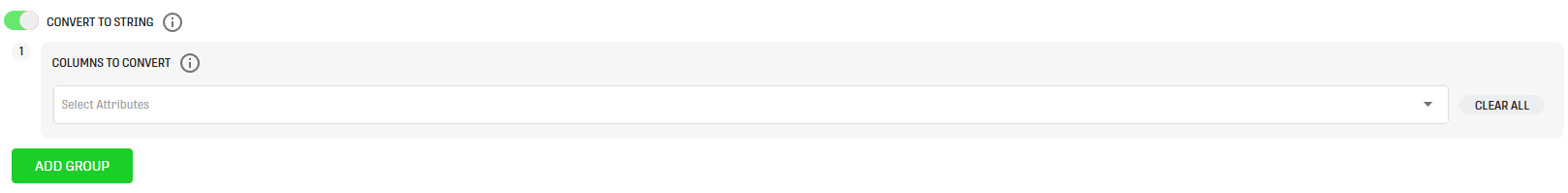All columns specified in this configuration are converted to text.

### Convert to DateTime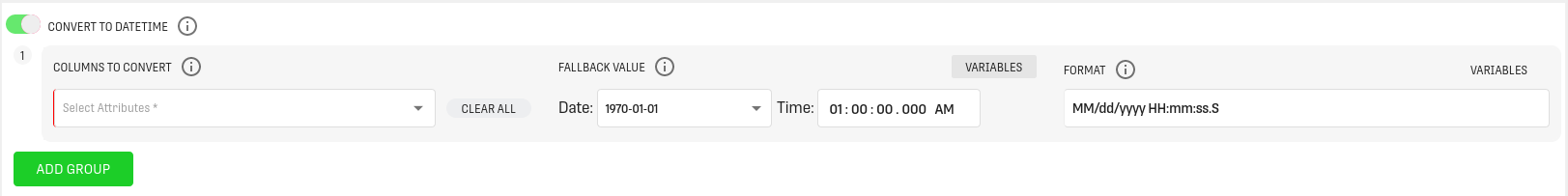With this configuration the values of the specified columns are converted to the datetime type, with the SPARK_TIMESTAMP format. Columns with string values are parsed with the given format, numeric values are treated as timestamps.

(More information about working with datetimes can be found here)

Options:

• Fallback Value: The value that is returned if the conversion of the input value fails. The datetime conversion also has an additional option for the fallback value. When clicking the "Variables" button, it is possible to select a workflow variable with type datetime as fallback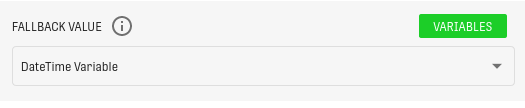• Format: Specify the datetime format as it is given in the selected columns. An example for a datetime format would be "M/d/y/ H:m:s:S". More information about datetime formats can be found here.
Note that the format option only applies to string values

### Convert to Double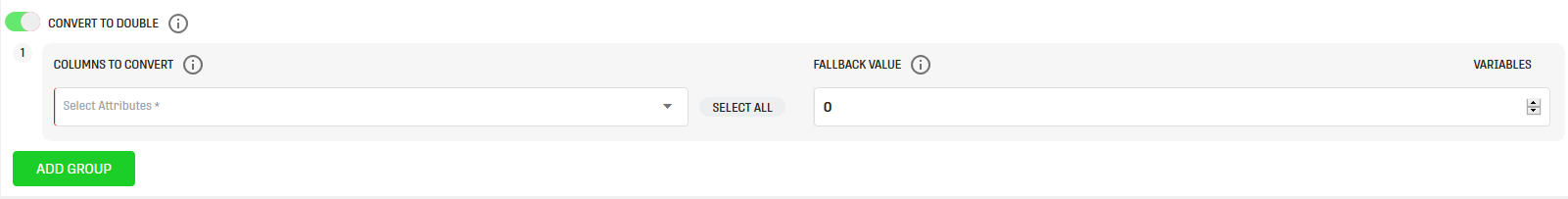With this configuration the values of the specified columns are converted to double. The input values need to be numerical.

Options:

• Fallback Value: Because the conversion returns double values, the fallback value has to be a number.

### Convert to Integer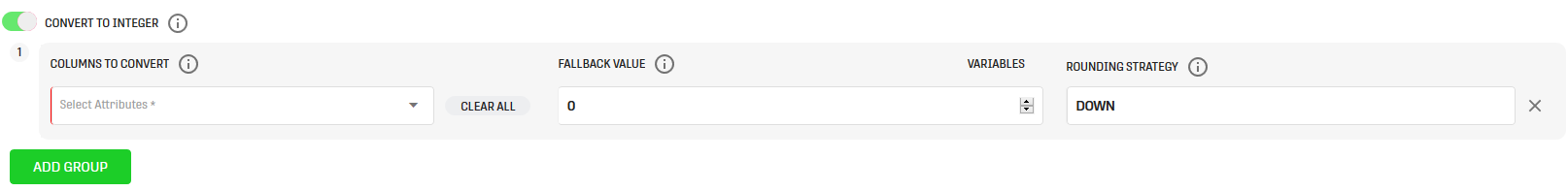With this configuration the values of the specified columns are converted to integer. Like in the previous configuration, the input values have to be a number.

Options:

• Fallback Value: Because the conversion returns integer values, the fallback value has to be a number.
• Rounding Strategy: Defines if and how converted numbers should be rounded. There are seven different strategies:
1. DOWN/CEILING: Rounds the input down (so it just drops the decimal places of the number)
2. UP/FLOOR: Rounds the input up to the next full number.
3. HALF_DOWN/HALF_UP: Rounds the decimal places of the input according to general rounding rules (from 0-4 down, from 5 - 9 up)

### General Information

It is also possible to add different conversion options for one type with the "Add Group" button. Imagine there are columns A and B with type double, that should be converted to integer, but with a different rounding strategy. So you can define the conversion for A, with rounding strategy "DOWN" and for B the rounding strategy "UP".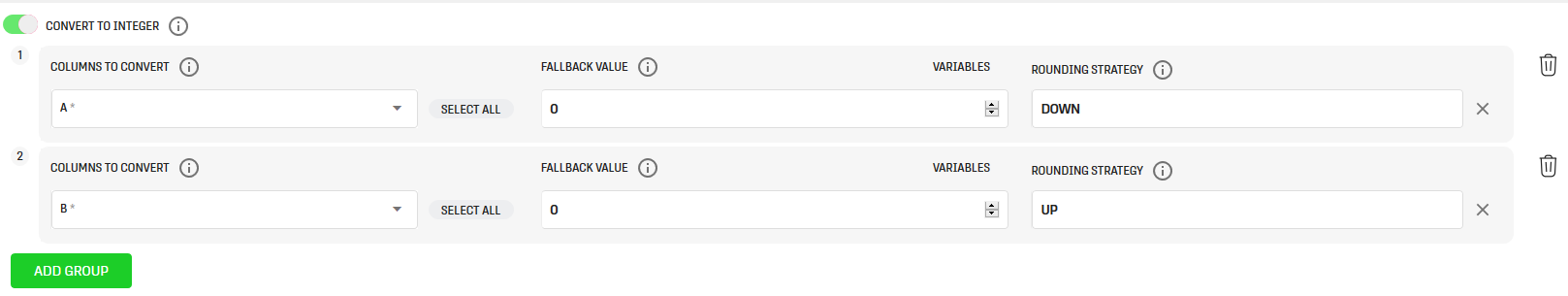# Output

The processor output contains the converted values for the specified columns. If the conversion failed for some values, the they now have the specified fallback value. Attributes that were not selected in the processor configuration remain the same.

# Example

## Example Input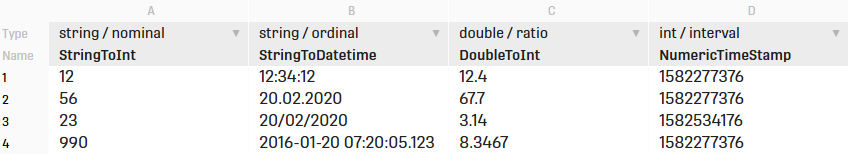Example Input Columns:

• StringToInt: Here we simply have some numbers represented as strings.
• StringToDatetime: Some datetimes in various formats represented as strings.
• DoubleToInt: This column contains some numbers represented as double.
• NumericTimeStamp: Here we have some numeric time stamps represented as integers.

## Example Configuration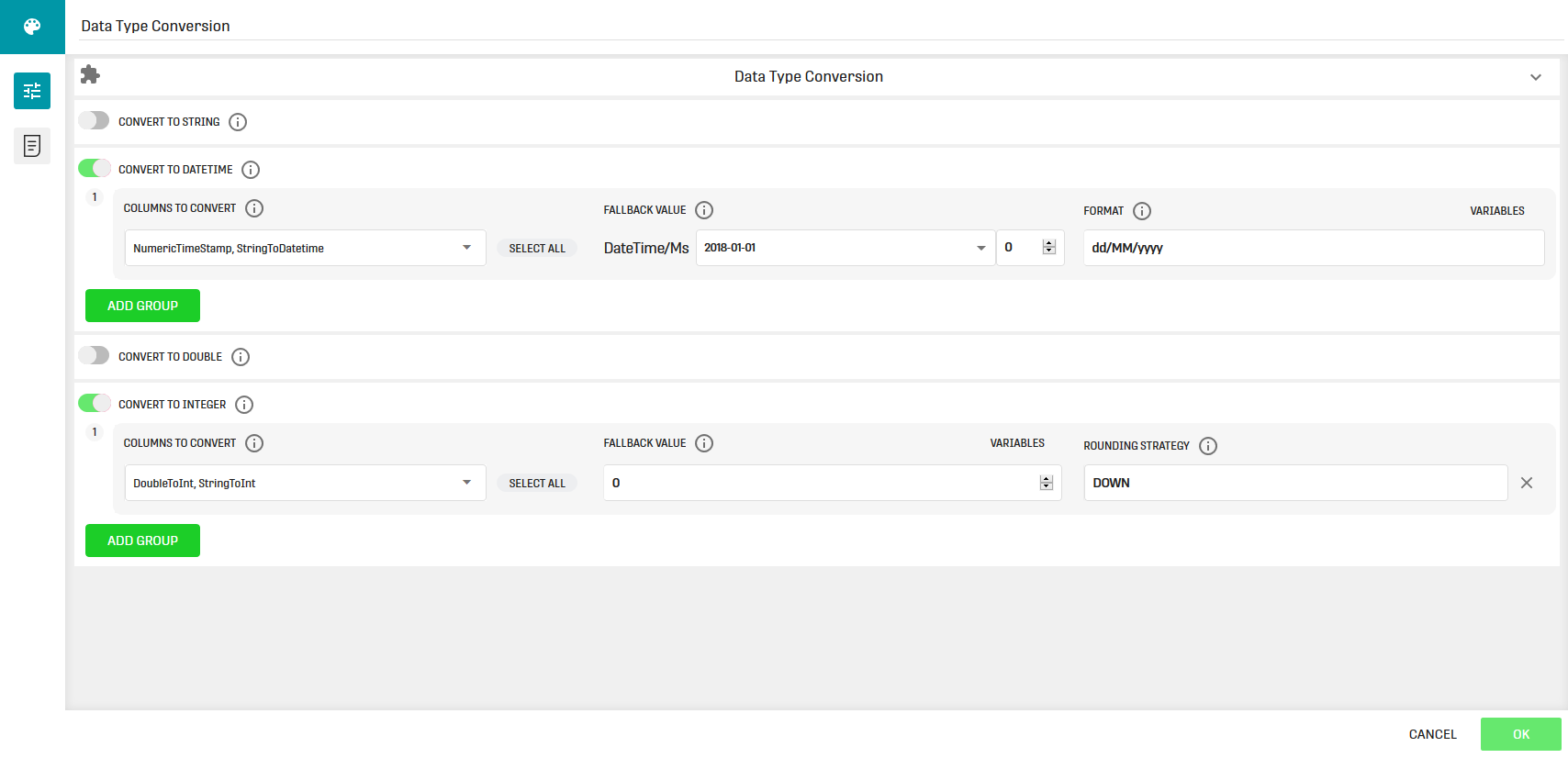## Result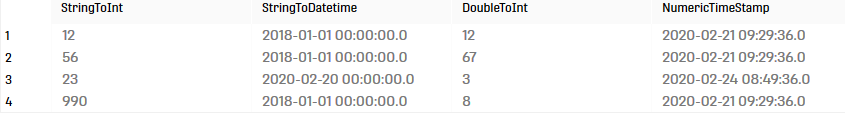Result Columns:

• StringToInt: The strings in the columns were converted to numbers.
• StringToDatetime: Here, only the third entry was correctly converted to datetime, the other rows contain the fallback value. This is because only the third row in the input matches the specified format from the configuration.
• DoubleToInt: The doubles were correctly converted to integer, also the specified rounding strategy "down" was applied.
• NumericTimeStamp: Here all the numeric timestamps were converted correctly to datetime.

Sometimes, it is not visible at first sight if the values were converted correctly, because Result Tables or Filterable Result Tables do not show the exact data type. If you want to check, open the "Json Result" View, and expand the schema tree. There are records that describe each column of the result, including data types.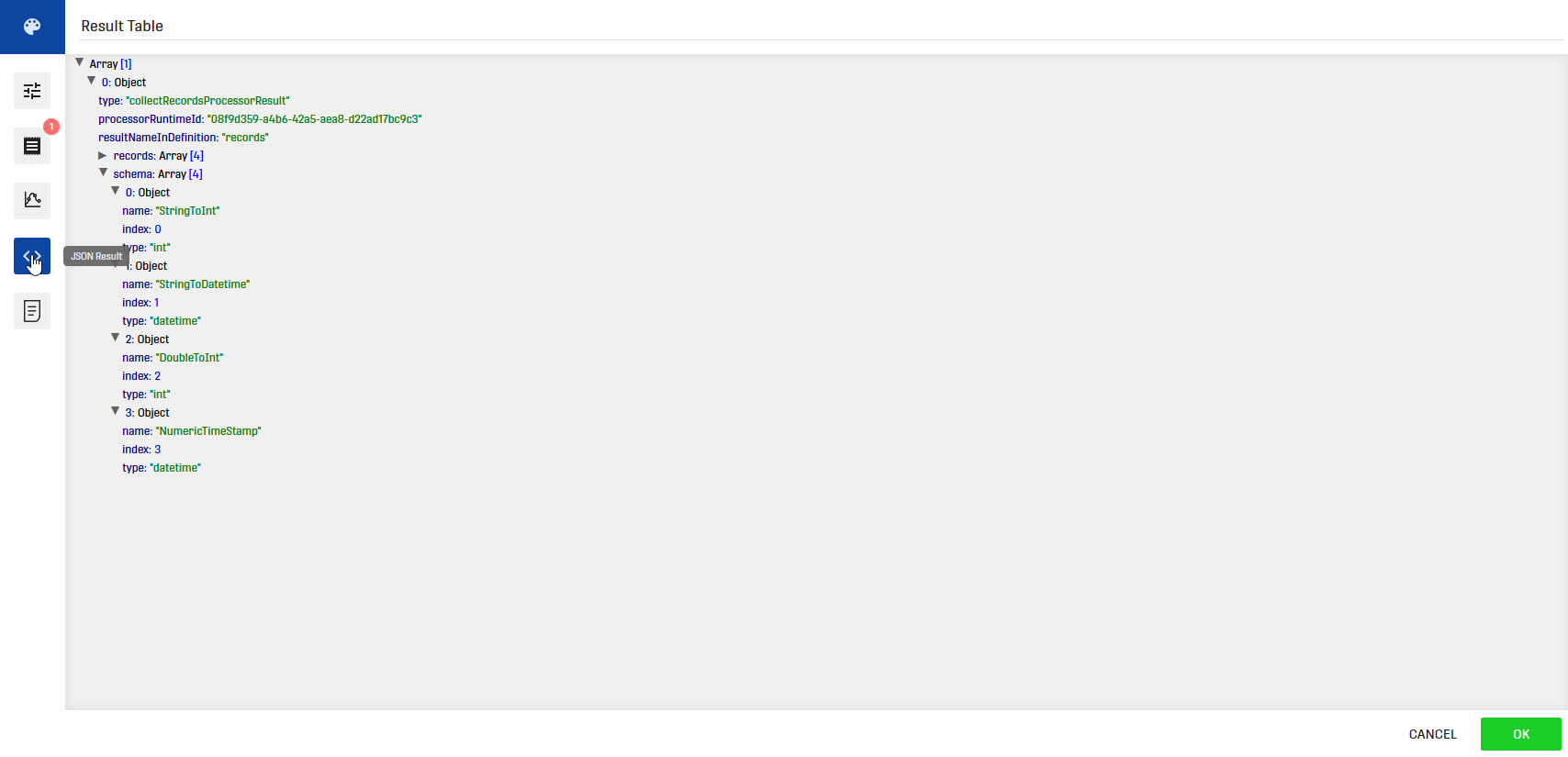# Related Articles

Data Type Recommendation Processor